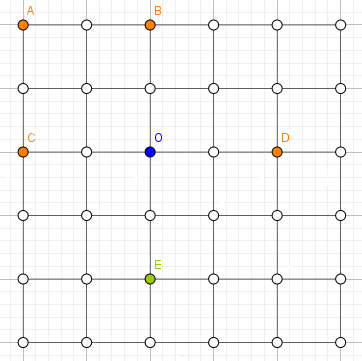# bzoj 1661: [Usaco2006 Nov]Big Square 巨大正方形 题解

### 1661: [Usaco2006 Nov]Big Square 巨大正方形

Time Limit: 5 Sec Memory Limit: 64 MB
Submit: 459 Solved: 224
[Submit][Status][Discuss]

#### Input

Line 1: 一个整数 N，2<=N<=100
Lines 2..N+1: 第 i+1 行描述点阵的第i行，有 N 个字符。字符集是： 'J' 表示这个点是农民 John 的牛， 'B'表示这个点是农民 Bob 的牛， '*' 表示这个点没有被占据。保证至少有一个点没有被占据。

#### Output

Line 1: 最大正方形的面积，或者无解的话输出0。

#### Sample Input

6
J*J***
******
J***J*
******
**B***
******


4

Silver

#### 题意：6的地图，橙色点为FJ的奶牛，绿色点为Bob的奶牛，白色点为未占用区域，能够成的最大正方形为 正方形ABOC ，面积为2x2=4。

### 题解A，B横坐标差=HG=HE=AE-AH；
A，B纵坐标差=AE+BF=AE+AH；

2DH=(A，B纵坐标差)+(A，B横坐标差)；
2AH=(A，B纵坐标差)-(A，B横坐标差)；

DH=CF=((A，B纵坐标差)+(A，B横坐标差))/2；
AH=BF=((A，B纵坐标差)-(A，B横坐标差))/2；
D点坐标即为(AX+DH,AY+AH);
C点坐标即为(BX-CF,BY-BF);L点坐标即为(JX+JP,JY+PL);
I点坐标即为(KX-NK,KY-NI);

#include<bits/stdc++.h>
#define F(i,j,n) for(register int i=j;i<=n;i++)
using namespace std;
int n,s=0,ans=0,a1,a2,a3,a4,a5,a6,a7,a8;
char a;
inline int read(){
int x=0,f=1;char ch=getchar();
while(ch<'0'||ch>'9'){if(ch=='-')f=-1;ch=getchar();}
while(ch>='0'&&ch<='9'){x=10*x+ch-'0';ch=getchar();}
return x*f;
}
inline int solve(int Sum,int Minus,char Kind){
if(Kind=='+')
return (Sum+Minus)>>1;
if(Kind=='-')
return (Sum-Minus)>>1;
}
int main(){
n=read();
F(i,1,n)
scanf("%s",a[i]+1);
F(i,1,n)/*枚举右下的点的横坐标*/
F(j,1,n)/*枚举右下的点的纵坐标*/
F(k,1,i)/*枚举左上的点的横坐标*/
F(tt,1,j){/*枚举左上的点的纵坐标*/
if(a[i][j]=='B'||a[k][tt]=='B')/*如果有Bob的牛就continue*/
continue;
int Sum=max(i-k,j-tt),Minus=min(i-k,j-tt);
if((Sum&1)!=(Minus&1))/*细节！判断奇偶性是否相同*/
continue;
int px=solve(Sum,Minus,'+'),py=solve(Sum,Minus,'-');/*计算出两式和与差*/
int p=k+px,q1=tt+py,u=i-px,v=j-py;/*得出剩下两点*/
if(((p-u)*(p-u)+(q1-v)*(q1-v))!=(Sum*Sum+Minus*Minus))/*考虑轴对称的情况*/
p=k+py,q1=tt+px,u=i-py,v=j-px;
if(p>=1&&q1>=1&&u>=1&&v>=1&&p<=n&&q1<=n&&u<=n&&v<=n)
if(a[i][j]!='B'&&a[k][tt]!='B'&&a[p][q1]!='B'&&a[u][v]!='B'){
s=0;
if(a[i][j]=='J')
s++;
if(a[k][tt]=='J')
s++;
if(a[p][q1]=='J')
s++;
if(a[u][v]=='J')
s++;
if(s>=3&&px*px+py*py>ans){
ans=px*px+py*py;
a1=i;a2=j;a3=u;a4=v;
a5=k;a6=tt;a7=p;a8=q1;
}
}
}
printf("%d\n",ans);
//printf("\n\n%d %d\n%d %d\n%d %d\n%d %d\n",a1,a2,a3,a4,a5,a6,a7,a8);
return 0;
}

posted @ 2018-08-11 21:39  hzf29721  阅读(246)  评论(0编辑  收藏  举报